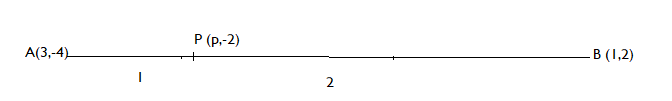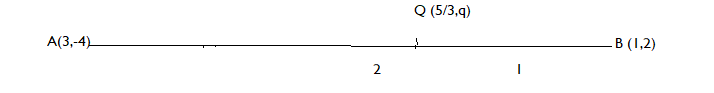QUESTION

# The line segment joining the points (3, -4) and (1,2) is trisected at the points P and Q. If the coordinates of P and Q are (p, -2) and ( $\dfrac{5}{3}$, q) respectively, find the values of p and q.A. p = $\dfrac{7}{3}$, q = – 3 B. p = $\dfrac{7}{3}$, q = 0C. p = $\dfrac{5}{3}$, q = 0 D. None of these

Hint: In this question to find the values of p and q, we will use the section formula and then check each given option to find out which are correct.

Now, we will use the section formula. The section formula tells us the coordinates of a point which divides a given line segment into two parts such that their lengths are in the ratio m: n. The Section formula to find a point (x, y) is

x = $\dfrac{{{\text{m}}{{\text{x}}_2}{\text{ + n}}{{\text{x}}_1}}}{{{\text{m + n}}}}$
y = $\dfrac{{{\text{m}}{{\text{y}}_2}{\text{ + n}}{{\text{y}}_1}}}{{{\text{m + n}}}}$

Here we are given two points. Let's say A (3, -4) and B (1,2) make a line segment AB. The line segment AB is trisected at points P and Q. So, we will apply section formula both at point P and Q. As, P divides line segment AB in ratio 1:2.So, applying section formula at point P (p, -2), we get
x – coordinate of P = $\dfrac{{1(1){\text{ + 2(3)}}}}{3}$ = $\dfrac{7}{3}$
$\Rightarrow$ p = $\dfrac{7}{3}$
As, Q divides the line segment AB in the ratio 2:1.Applying section formula at point Q ( $\dfrac{5}{3}$, q), we get
y – coordinate of Q = $\dfrac{{2(2){\text{ + 1( - 4)}}}}{3}$ = 0
$\Rightarrow$ q = 0
So, the correct option will be B.

Note: While solving such types of questions, it is important to apply the section formula correctly. Most of the students made a mistake while applying the section formula. They replaced m from n and n from m. Also, apply the proper ratio for finding the coordinates of a point. Also, when only you have to find the value of a given variable, apply only that formula to find the value of the variable, for example if you are given that the x – coordinate of point is k, so you have to apply only the formula to find the x – coordinate of point.